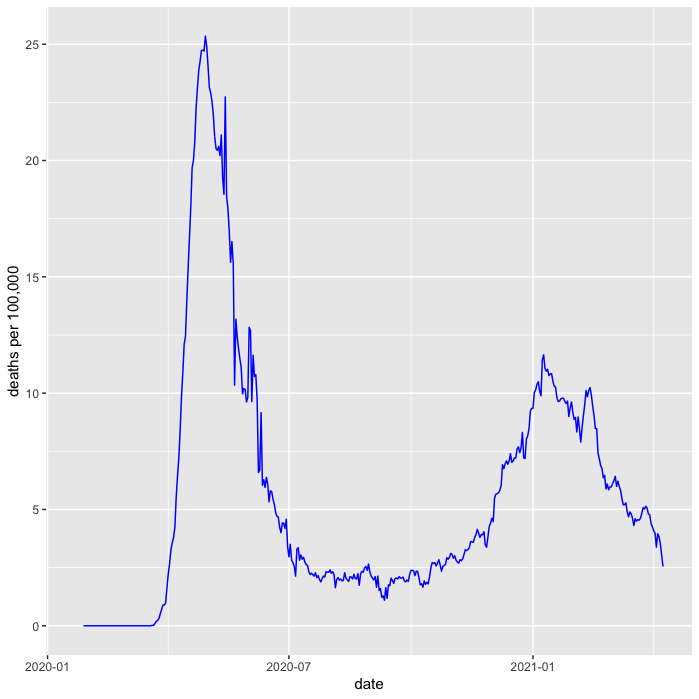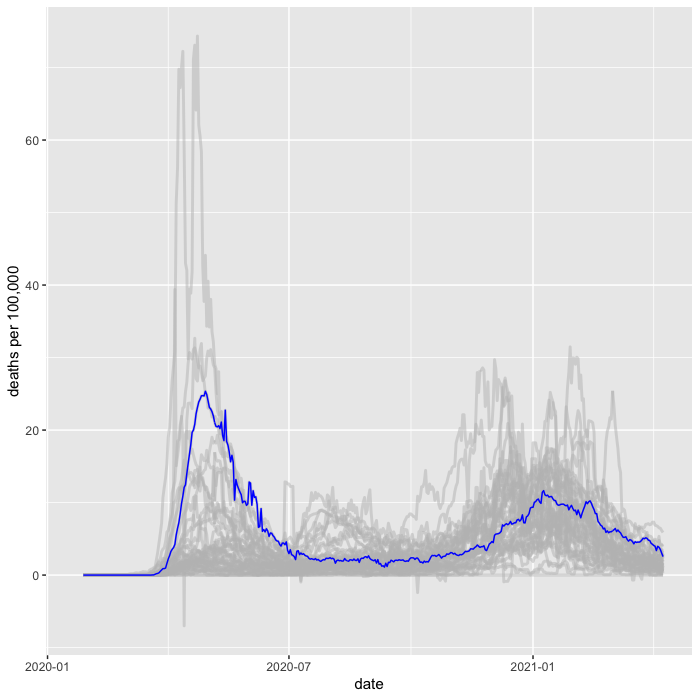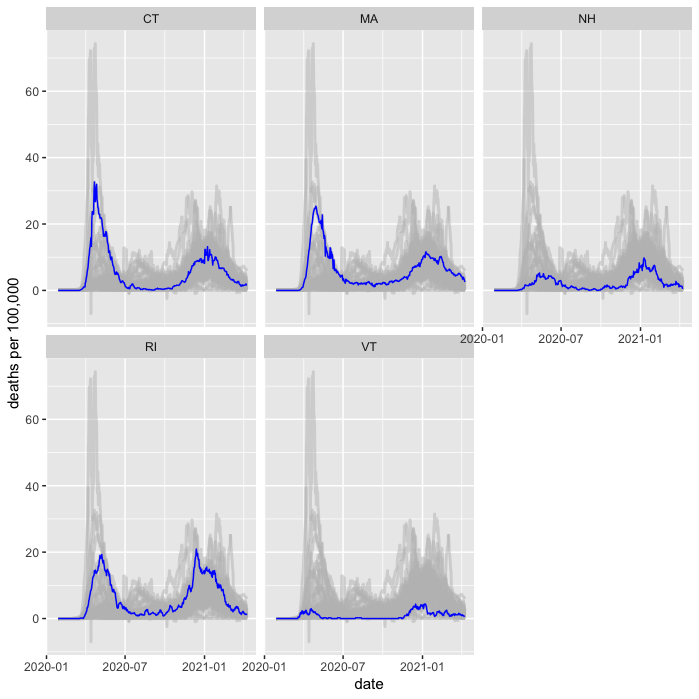# Muted spaghetti line charts with R's ggplot2

12 April 2021

If someone tells me their sales last month was \$10M - what do I make of it? With just the bare number, I don't know what to think. To make sense of the number I need context, perhaps over time, perhaps compared to compatible companies. Using a data visualization can help me put a number into a context that allows me to make sense of it.

One particularly useful form of context is context over time. How does today's figure match up with that value over time? A line chart, plotted against time, helps me see this.

Here is a rather more sombre example than sales revenues, the deaths per 100,000 due to covid in the state of Massachusetts.This is valuable, as I can now put today's figure in historical context, comparing recent figures to those in the last two peaks. It's also very easy to plot this chart in R, needing just a few lines of code.

```death_pp %>%
filter(state == "MA") %>%
ggplot(aes(date, death_pm_rm)) +
labs(y = "deaths per 100,000") +
geom_line(color = "blue")
```
```# cdc covid data records New York City seperately from New York state
cdc_pops <- pops %>%
mutate(pop = if_else(state == "NY", pop - 8400000, pop)) %>%
add_row(name = "New York City", state = "NYC", pop = 8400000)

# http  -d "https://data.cdc.gov/api/views/9mfq-cb36/rows.csv" > cdc_cases.csv
select(state, submission_date, new_death, tot_death) %>%
mutate(date = mdy(submission_date)) %>%
arrange(date) %>%
group_by(state)

death_pp <- cdc_cases %>%
left_join(cdc_pops, by = "state") %>%
drop_na(pop) %>%
mutate(death_pm = new_death * 1000000 / pop) %>%
mutate(death_pm_rm = rollmean(death_pm, 7, fill=NA, align="right"))
```

But I can show more context than just time. To better understand how the epidemic has been in Massachusetts, I can compare it to how things have gone in the other states. A good way to do this is to show the line chart for every other US state as a muted background.As far as I can tell, there's no generally accepted term for this kind of plot. Putting multiple lines on a line chart is sometimes referred to as a spaghetti line chart. So I'll refer to this as a muted-spaghetti chart.

In R it's pretty easy to plot this, the key is to plot another `geom_line` with a different data source as the primary line we're looking at.

```death_pp %>%
filter(state == "MA") %>%
ggplot(aes(date, death_pm_rm)) +
labs(y = "deaths per 100,000") +
geom_line(data = death_pp, aes(group = state), color = "grey", size = 1, alpha = 0.5) +
geom_line(aes(y = death_pm_rm), color = "blue")
```

Note that I plot the background before the foreground line to ensure the foreground line pops clearly on top.

## Doing this with a grid (facets)

Showing this with one state is good, but it's often useful to be able to look at several states in this way. `ggplot2` provides the very nifty `facet_wrap` command to plot a line chart for every value in a set, but it requires a little trickery to make it work with a muted-spaghetti background like this.The trickery comes with the way I need to specify the grouping for the spaghetti.

```death_pp %>%
filter(state %in% c("MA", "VT", "CT", "RI", "NH")) %>%
ggplot(aes(date, death_pm_rm)) +
labs(y = "deaths per 100,000") +
geom_line(data = death_pp %>% rename(s = state),
aes(group = s), color = "grey", size = 1, alpha = 0.5) +
geom_line(color = "blue") +
facet_wrap(~state, ncol = 3)
```

By renaming the grouping column, ggplot only facets the primary line and plots the spaghetti on each facet. 

## Footnotes

1: It took me ages of experimenting and web searching to find how to do this with facets. Eventually I found the answer at from data to viz